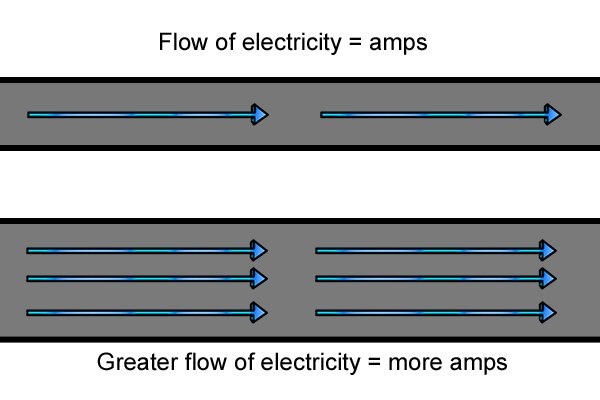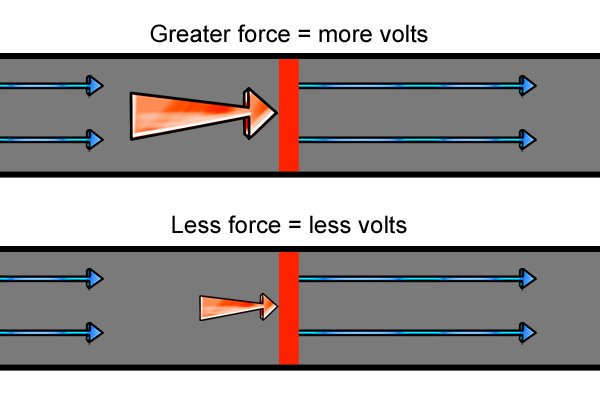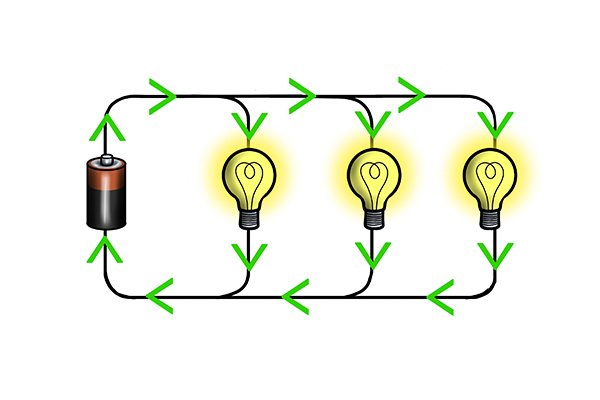# What are amps, volts and watts?

Shop for Batteries and ChargersAmps, volts and watts are all used to describe electricity and are very important when choosing a cordless power tool battery or charger but if you haven’t come across these terms before it can be confusing at first.Imagine electricity flowing through a wire. Amps describe how much electricity can flow through the wire, this is known as the ‘electrical current’. If not much electricity can flow through the wire then the amps are a low number; if lots of electricity can flow then the amps are a high number.Volts describe how much force, or ‘electrical pressure,’ is pushing the electricity through the wire. This is also known as ‘voltage’. Increasing the pressure of the electricity increases the number of volts.Watts describe how much work the electricity can do, for example, how long it can keep a light on for. This is known as the ‘electrical power’. Increasing the volts or increasing the amps will increase the number of watts or ‘wattage.’ The mathematical equation is:

Watts = Voltage x Current

Call Now Button